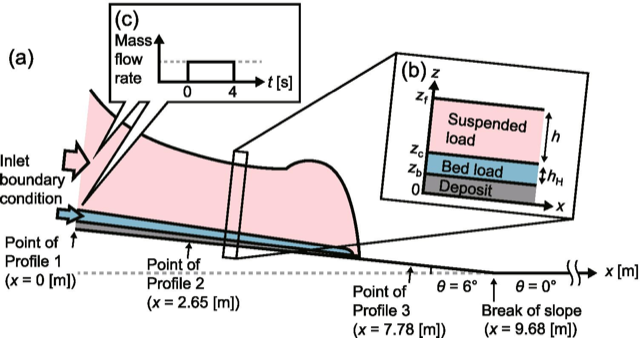Sei qui: Home > Archiviati > Validation of a two‑layer depth‑averaged model by comparison with an experimental dilute stratified pyroclastic density current

## Validation of a two‑layer depth‑averaged model by comparison with an experimental dilute stratified pyroclastic density currentSchematic illustration of a series of numerical simulations of the two-layer PDC model. a A two-layer PDC is generated from the inlet boundary (x=0) and flows into a proximal inclined channel with length 9.68 m (slope angle θ=6∘) and into a distal horizontal channel (θ=0∘). b There are three interfaces, between the upper suspended load with thickness h and the ambient air (z=zf), between the lower bed load with thickness hH and the suspended load (z=zc), and between the deposit and the bed load (z=zb). c The inlet mass flow rates of the suspended and bed loads are given as constant values for time t=0–4 s and as 0 for t>4 s

Shimizu H. A., T. Koyaguchi, Y.J. Suzuki, E. Brosch, G. Lube, & M. Cerminara (2021).
Bulletin of Volcanology, doi:10.1007/s00445-021-01493-w

### Abstract

Numerical results of a two-layer depth-averaged model of pyroclastic density currents (PDCs) were compared with an experimental PDC generated at the international eruption simulator facility (the Pyroclastic flow Eruption Large-scale Experiment (PELE)) to establish a minimal dynamical model of PDCs with stratification of particle concentrations. In the present two-layer model, the stratification in PDCs is modeled as a voluminous suspended-load layer with low particle volume fractions (≲10−3) and a thin basal bed-load layer with higher particle volume fractions (∼10−2) on the basis of the source condition in the experiment. Numerical results for the suspended load quantitatively reproduce the time evolutions of the front position and flow thickness in the experimental PDC. The numerical results of the bed-load and deposit thicknesses depend on an assumed value of settling speed at the bottom of the bed load (WsH). We show that the thicknesses of bed load and deposit in the simulations agree well with the experimental data, when WsH is set to about 1.25×10−2 m/s. This value of the settling speed is two orders of magnitude smaller than that predicted by a hindered-settling model. The small value of WsH is considered to result from decreasing in the effective deposition speed due to the erosion process accompanied by saltating/rolling of particles at the bottom of the bed load.